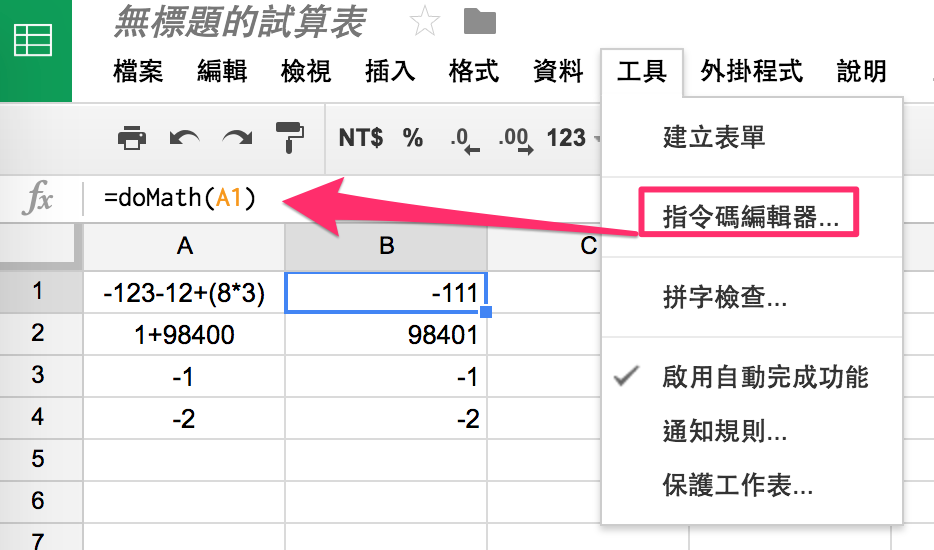儲存格 Evaluation 的使用情境

1. 匯款花了台幣 1014 元。如果直接寫 1014 元我事後無從得知這筆費用是否包含手續費。而如果寫的是 1000+14 很清楚地知道這筆費用已涵蓋了手續費。
2. 出差花了人民幣 100 元。如果寫的是 100*4.89，我們就可以很清楚看出當日匯率是 4.89，花的是人民幣不是台幣。

Excel 是支援儲存格內運算的，不過必須填入 =1000+14 而不是 1000+14。這樣的寫法就會讓算式不可讀，只剩下運算後的結果，每筆費用明細需要到各自的儲存格才能查看。

Excel 上的 Evaluation 方式

1. 首先在「公式」的頁籤中定義名稱。翻譯可能有點混淆，這裡指的名稱是公式的名稱。
2. 定義好名稱之後選定你的儲存格，作為 EVALUTE 的函數參數輸入
3. 在儲存格的內容填上剛剛定義好的公式名稱 =doMatchfunction doMath( formula ) {
// Strip leading "=" if there
if (formula.charAt(0) === '=')
formula = formula.substring(1);
return eval(formula)
}

1. 開啟指令編輯器
2. 貼上程式碼
3. 回到試算表中
4. 直接使用剛剛在編輯器中定義好的函數 doMathfunction doMath( formula ) {
Logger.log(sheet.getRange('A1').getValue());

// Strip leading "=" if there
if (formula.charAt(0) === '=')
formula = formula.substring(1);
return eval(formula)
}